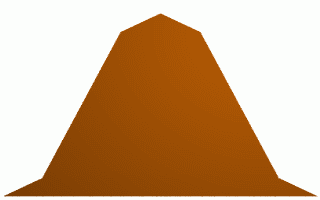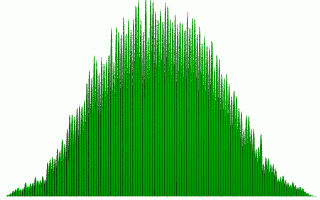Previous Contents Next Mass Scenes Rendering Framework page 3 of 8

3. Parametric object modifications

The basic support for parametric object description comes from the standard POV-Ray's scene description language. The workflow of POV-Ray image creation is: create the scene source file (a text file), run the parser (that creates internal rendering structures - the scene), start the rendering engine. This allows the straightforward design of parametric objects using macros. A macro represents the objects to be generated many times in the scene. To let them all have different color, the only task is to add the color choice to the macro. The object's macro is parsed several times and each time a different color is chosen.

3.1 Random function

The production of large amounts of different objects cannot be done without a good random function. In POV-Ray there is a random function that generates a random number in the range between zero and one with constant density function; that means each number has the same probability to be chosen. In the real life no measurable parameter of almost any object has such distribution . The basic distribution is the so-called normal distribution, where the density function is the gaussian curve. If the parametric definition of a 3D character requires to generate a number representing the figure height, it is much better to use a random number generated with the normal distribution instead of the standard random function. Maybe there is no reason to do such (a bit more complicated) calculation during the generation of only one instance of the object, but it is a real requirement for mass scenes.

What if the scene author wants to have a few small persons and a lot of tall ones? The normal distribution cannot be used. Still there is no reason why the author should not enter the density function that satisfies his intention. That is exactly the first extension of the POV-Ray scene description language - a function that generates a random value according to a given density function and its placement (the centre and the dispersion).

To enter the data describing the density function into the POV-Ray scene description language, an array comes to good use. The values in the array represent the density function ordinates, the abscises are equidistant. Two examples are given - a constant density function, and a gaussian-like density function:

#declare constant_density = array {1.0, 1.0}
#declare gaussian_density = array
{0.0, 0.1, 0.5, 0.9, 1.0, 0.9, 0.5, 0.1, 0.0}

The code requesting a random value having the gaussian density function (according to the above example) applied to the height of a human - centred to 170 centimetres and with the dispersion of 30 cm will look similar to this one:

#declare height = makevalue(gaussian_density,
170.0, 30.0, random_stream);Figure 1: The gaussian density function data. Figure 2: The makevalue function empirical data.

Where could the generated numbers be used? Anywhere - for sizes of the objects, for color values, for position coordinates, for pattern modifications, anywhere where a random value is useful.

3.2 Alternative

The second randomization of the macro representing the parametric object comes from the need to make decisions. Suppose, (for instance) that it is needed to decide whether a person will wear short or long trousers. Perhaps it is possible to describe the trousers length by the makevalue function with proper settings, but if there is the need to make totally different objects for the trousers, it has to be determined which type of the trousers to use. An alternative directive is the second contribution to the POV-Ray scene description language.

Usually the scene designer has the feeling of the percentage - the partial amounts of how many of the generated objects should comply a given condition. In the above example it could be stated that (for example) sixty percent of the people would have long trousers, and forty percent the short ones. An alternative directive added into the macro could express it in this way:

#alternative (random_value)
#case (0.60) longTrousersMacro()
#case (0.40) shortTrousersMacro()
#end

The alternative could be used wherever a decision has to be applied - for color selection, for the choice of object type, to choose any optional orientation. It is advantageous to use the alternative in the macro of the generated object to determine which object should be actually generated. A typical example occurs while modelling a common food - a letter soup, where the soup contains different soup elements:

#macro soupElement()
#case (0.86) letter()
#case (0.08) carrot()
#case (0.06) parsley()
#end

Mass Scenes Rendering Framework, Dušan Bezák, 1999-2001, http://www.ksp.sk/~dushan/dipl/